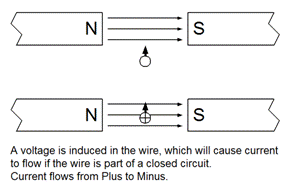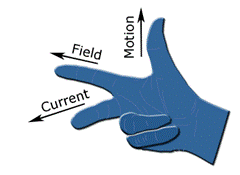Experiments with magnets and our surroundings

Twelve Fundamentals of Magnetism

10.   Generator Action

http://en.wikipedia.org/wiki/Electric_generator
b. What does this mean?

When a wire and magnetic field move perpendicularly with respect to each other, a voltage is induced (or generated) within the wire.
The induced voltage then causes current to flow within the wire if it is part of a closed circuit.This phenomenon was discovered by Michael Faraday in 1831 and Joseph Henry in 1832, independently of each other.

d. How much voltage is induced (generated)?

The magnitude of the induced voltage, Eg = B*L*v

Where:
i. Eg is the induced (generated) voltage, Volts

ii. B is the magnetic flux density, T, within which the wire is moving

iii. L is the length of the wire in meters which is immersed within the magnetic flux density

iv. v is the velocity of the wire, m/sec, perpendicular to the magnetic flux

A very simple equation!  The voltage induced is only affected by the strength of the magnetic field, how fast the wire is moving, and the length of the wire within the field.

If the wire is moving at an angle other than 90 to the magnetic field, the induced voltage will be multiplied by the sine of the angle.

So, a voltage will be present between the two ends of the wire coming from the generator, even if no current is flowing.

e. What will be the polarity of the induced voltage (what direction will the current flow)?

The polarity of the voltage, and the direction of the current in the wire, can be determined using the Right-Hand-Rule (RHR)

i. The thumb of the right hand points in the direction of motion of the wire with respect the magnetic flux

ii. The first (index) finger of the right hand points in the direction of the magnetic flux (or think of this as the first finger pointing in the direction of the field)

iii. The second finger of the right hand points in the direction of the current that would flow due to the induced (generated) voltage.